# NCERT Solutions - Atoms and Molecules, Science, Class 9 Class 9 Notes | EduRev

## Class 9 : NCERT Solutions - Atoms and Molecules, Science, Class 9 Class 9 Notes | EduRev

The document NCERT Solutions - Atoms and Molecules, Science, Class 9 Class 9 Notes | EduRev is a part of the Class 9 Course Class 9 Science by VP Classes.
All you need of Class 9 at this link: Class 9

Q1. In a reaction 5.3 g of sodium carbonate reacted with 6 g of acetic acid. The products were 2.2 g of carbon dioxide, 0.9 g water and 8.2 g of sodium acetate. Show that these observations are in agreement with the law of conservation of mass carbonate.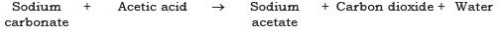Ans.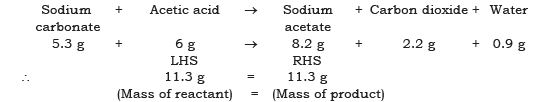This shows that during a chemical reaction mass of reactant = mass of product.

Q2. Hydrogen and oxygen combine in the ratio of 1 : 8 by mass to form water. What mass of oxygen gas would be required to react completely with 3 g of hydrogen gas?
Ans.
Ratio of H : O by mass in water is: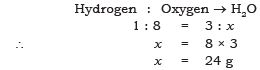∴ 24 g of oxygen gas would be required to react completely with 3g of hydrogen gas.

Q3. Which postulate of Dalton’s atomic theory is the result of the law of conservation of mass?
Ans.
The postulate of Dalton’s atomic theory that is the result of the law of conservation of mass is—the relative number and kinds of atoms are constant in a given compound. Atoms cannot be created nor destroyed in a chemical reaction.

Q4. Which postulate of Dalton’s atomic theory can explain the law of definite proportions?
Ans.
The relative number and kinds of atoms are constant in a given compound.

Q5. Define the atomic mass unit.
Ans.
One atomic mass unit is equal to exactly one-twelfth (1/12th) the mass of one atom of carbon-12. The relative atomic masses of all elements have been found with respect to an atom of carbon-12.

Q6. Why is it not possible to see an atom with naked eyes?
Ans.
Atom is too small to be seen with naked eyes. It is measured in nanometres. 1 m = 109 nm

Q7. Write down the formulae of
(i) Sodium oxide
(ii) Aluminium chloride
(iii) Sodium sulphide
(iv) Magnesium hydroxide
Ans. The formulae are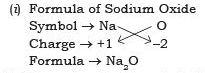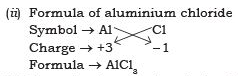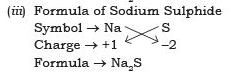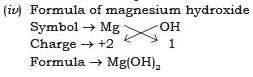Q8. Write down the names of compounds represented by the following formulae:
(i) Al2(SO4)3 (ii) CaCl2 (iii) K2SO4 (iv) KNO3 (v) CaCO3.
Ans.
(i) Al2(SO4)3 → Aluminium sulphate
(ii) CaCl2 → Calcium chloride
(iii) K2SO4 → Potassium sulphate
(iv) KNO3 → Potassium nitrate
(v) CaCO3 → Calcium carbonate

Q9. What is meant by the term chemical formula?
Ans.
The chemical formula of the compound is a symbolic representation of its composition, e.g., chemical formula of sodium chloride is NaCl.

Q10. How many atoms are present in a (i) H2S molecule and (ii) PO43– ion?
Ans.
(i) H2S → 3 atoms are present, 2 atoms of hydrogen and 1 atom of sulphur
(ii) PO43– → 5 atoms are present, 1 atom of phosphorus and 4 atoms of oxygen.

Q11. Calculate the molecular masses of H2, O2, Cl2, CO2, CH4, C2H6, C2H4, NH3, CH3OH.
Ans.
The molecular masses are:

H⇒ 1 X 2 = 2U
O2 ⇒ 16 X 2 =  32 U
Cl2  ⇒ 35.5 X 2 = 71 U
CO2  ⇒  1 X 12+2 X 16 = 12 +32 = 44 U
CH4  ⇒ 1 X 12 +4 X 1 = 16 U
C2H6  ⇒ 2 X 12 + 6 X 1 = 30U
C2H4  ⇒ (2 X 12) + (4 X 1) = 28U
NH3  ⇒ (1 X 14) + (3 X 1) = 17U
CH3OH ⇒ 12 + (3 X 1) + 16 + 1 = 32 U

Q12. Calculate the formula unit masses of ZnO, Na2O, K2CO3, given atomic masses of Zn = 65 u, Na = 23 u, K = 39 u, C = 12 u, and O = 16 u.
Ans.
The formula unit mass of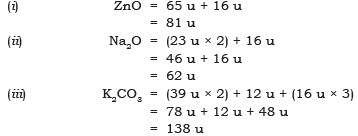Q13. If one mole of carbon atoms weigh 12 grams, what is the mass (in grams) of 1 atom of carbon?
Ans.
1 mole of carbon atoms = 6.022 × 1023 atoms = 12 g Mass of 1 atom = ?

∴ Mass of 1 atom of carbon  =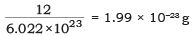Q14. Which has more number of atoms, 100 grams of sodium or 100 grams of iron (given atomic mass of Na = 23 u, Fe = 56 u)?
Ans
. 23 g of Na = 6.022 × 1023 atoms (1 mole).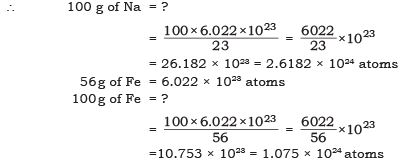100 g of Na contain→ 2.618 × 1024 atoms
100 g of Fe contain→ 1.075 × 1024 atoms
∴ 100g of Na contains more atoms.

NCERT TExTUAL QUESTIONS SOLVED

Q1. A 0.24 g sample of compound of oxygen and boron was found by analysis to contain 0.096 g of boron and 0.144 g of oxygen. Calculate the percentage composition of the compound by weight.
Ans
. Boron and oxygen compound → Boron  + Oxygen
0.24 g → 0.096 g + 0.144 g
Percentage composition of the compound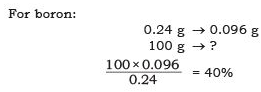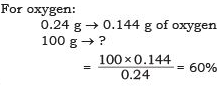Q2. When 3.0 g of carbon is burnt in 8.00 g oxygen, 11.00 g of carbon dioxide is produced. What mass of carbon dioxide will be formed when 3.00 g of carbon is burnt in 50.00 g of oxygen? Which law of chemical combination will govern your answer?
Ans.
The reaction of burning of carbon in oxygen may be written as: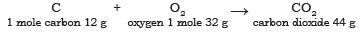It shows that 12 g of carbon burns in 32 g oxygen to form 44 g of carbon dioxide. Therefore 3 g of carbon reacts with 8 g of oxygen to form 11 g of carbon dioxide. It is given that 3.0 g of carbon is burnt with 8 g of oxygen to produce 11.0 g of CO2. Consequently 11.0 g of carbon dioxide will be formed when 3.0 g of C is burnt in 50 g of oxygen consuming 8 g of oxygen, leaving behind 50 – 8 = 42 g of O2. The answer governs the law of constant proportion.

Q3. What are polyatomic ions? Give examples.
Ans.
The ions which contain more than one atoms (same kind or may be of different kind) and behave as a single unit are called polyatomic ions e.g., OH, SO42–, CO32-.

Q4. Write the chemical formulae of the following:
(a) Magnesium chloride
(b) Calcium oxide
(c) Copper nitrate
(d) Aluminium chloride
(e) Calcium carbonate.

Ans.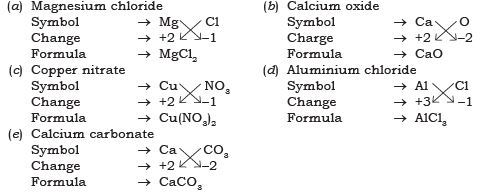Q5. Give the names of the elements present in the following compounds:
(a) Quick lime
(b) Hydrogen bromide
(c) Baking powder
(d) Potassium sulphate.
Ans.
(a) Quick lime → Calcium oxide Elements → Calcium and oxygen
(b) Hydrogen bromide Elements → Hydrogen and bromine
(c) Baking powder → Sodium hydrogen carbonate Elements →     Sodium, hydrogen, carbon and oxygen
(d) Potassium sulphate Elements →     Potassium, sulphur and oxygen

Q6. Calculate the molar mass of the following substances.
(a) Ethyne, C2H2
(b) Sulphur molecule, S8
(c) Phosphorus molecule, P4 (Atomic mass of phosphorus = 31)
(d) Hydrochloric acid, HCl
(e) Nitric acid, HNO3
Ans.
The molar mass of the following: [Unit is ‘g’]
(a) Ethyne, C2H2 = (2 × 12) + (2 × 1) = 24 + 2 = 26 g
(b) Sulphur molecule, S8 = 8 × 32 = 256 g
(c) Phosphorus molecule, P4 = 4 × 31 = 124 g
(d) Hydrochloric acid, HCl = (1 × 1) + (1 × 35.5)
= 1 + 35.5 = 36.5 g
(e) Nitric acid, HNO3= (1 × 1) + (1 × 14) + (3 × 16)
= 1 + 14 + 48 = 63 g

Q7. What is the mass of
(a) 1 mole of nitrogen atoms?
(b) 4 moles of aluminium atoms (Atomic mass of aluminium = 27)?
(c) 10 moles of sodium sulphite (Na2SO3)?
Ans.
(a) Mass of 1 mole of nitrogen atoms = 14 g

(b) 4 moles of aluminium atoms Mass of 1 mole of aluminium atoms = 27 g
∴ Mass of 4 moles of aluminium atoms = 27 × 4 = 108 g

(c) 10 moles of sodium sulphite (Na2SO3)
Mass of 1 mole of Na2SO3 = (2 × 23) + 32 + (3 × 16) = 46 + 32 + 48 = 126 g
∴     Mass of 10 moles of Na2SO3 = 126 × 10 = 1260 g

Q8. Convert into mole. (a) 12 g of oxygen gas (b) 20 g of water (c) 22 g of Carbon dioxide.
Ans.
(a) Given mass of oxygen gas = 12 g Molar mass of oxygen gas (O2) = 32 g
∴ Mole of oxygen gas = (12/32)=0.375 mole

(b) Given mass of water = 20 g Molar mass of water (H2O) = (2 × 1) + 16 = 18 g
∴ Mole of water = 1280 =  1.11 mole

(c) Given mass of Carbon dioxide = 22 g
Molar mass of carbon dioxide (CO2) = (1 × 12) + (2 × 16) = 12 + 32 = 44g
∴ Mole of carbon dioxide = (22/44)= 0.5 mole

Q9. What is the mass of:
(a) 0.2 mole of oxygen atoms? (b) 0.5 mole of water molecules?
Ans.
(a) Mole of Oxygen atoms = 0.2 mole Molar mass of oxygen atoms = 16 g
∴ Mass of oxygen atoms = 16 × 0.2 = 3.2 g

(b) Mole of water molecule = 0.5 mole Molar mass of water molecules = (2 × 1) + 16 = 18 g
∴ Mass of H2O = 18 × 0.5 = 9 g

Q10. Calculate the number of molecules of sulphur (S8) present in 16 g of solid sulphur.
Ans.
Molar mass of S8 sulphur = 256 g = 6.022 × 1023 molecules Given mass of sulphur = 16 g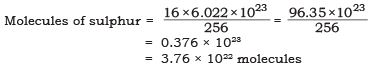Q11. Calculate the number of aluminium ions present in 0.051 g of aluminium oxide.
(Hint: The mass of an ion is the same as that of an atom of the same element. Atomic mass of Al = 27 u)
Ans.
Molar mass of aluminium oxide Al2O3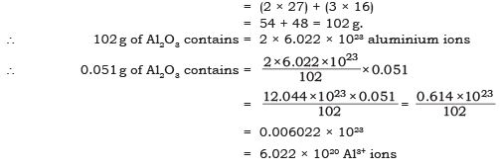Offer running on EduRev: Apply code STAYHOME200 to get INR 200 off on our premium plan EduRev Infinity!

75 docs|14 tests

,

,

,

,

,

,

,

,

,

,

,

,

,

,

,

,

,

,

,

,

,

,

,

,

,

,

,

;